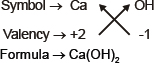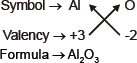## Atoms and Molecules:

Have you ever wondered about the smallest particle existing in the whole universe? Although, you can’t see them with the naked eye but you can think about them. They are called as atoms. Atoms are also known as building blocks of all matter. This has been discovered that atoms are also divisible and can be further divided into electrons, protons, and neutrons, which further can be subdivided into quarks.  In short, everything you see around yourself is made of atoms, even you yourself are made up of atoms. This sounds interesting, isn’t it? So, now you might be thinking about the size of an atom as I said they are too small rather smallest that their radii are measured in nanometres (10-9 meter). Atoms mostly can’t exist independently, they exist in the form of molecules or ions. These molecules aggregate in large numbers to form matter. So, a molecule is a group of two or more atoms chemically bonded together. In this article, find everything you need to know about atoms and molecules!

Now, when you know about atoms and molecules, shouldn’t we discuss some theories, laws, and terms related to them?

## Laws of Chemical Combination:

### 1. Law of Conservation of Mass:

This law states that during a chemical reaction, the total mass of products will be equal to the total mass of reactants i.e. mass can neither be created nor be destroyed.

Eg: A(Reactant) + B(Reactant) = AB(Product)

mass of A + mass of B = mass of AB

### 2. Law of Constant Proportions:

In a chemical substance, elements are always present in definite proportions by mass. This law was given by Lavoisier.

Eg: In water (H2O), the ratio of Hydrogen(H) to Oxygen(O) by mass is always 1:8.

These laws lacked adequate explanation. Hence, John Dalton formulated his Atomic Theory which provided an explanation for these laws.

## Dalton’s Atomic Theory:

According to Dalton’s atomic theory, all matter, whether an element, a compound or a mixture is composed of small particles called
atoms.

The postulates of his theory can be stated as follows:

(i) All matter is made of very tiny particles called atoms.
(ii) Atoms are indivisible particles, which cannot be created or destroyed in a chemical reaction.
(iii) Atoms of a given element are identical in mass and chemical properties.
(iv) Atoms of different elements have different masses and chemical properties.
(v) Atoms combine in the ratio of small whole numbers to form compounds.
(vi) The relative number and kinds of atoms are constant in a given compound.

### Symbols of Chemical Elements:

Dalton proposed some symbols for chemical elements by which he meant the specific quantity of an element i.e. one atom of an element.Symbols of elements as proposed by Dalton

Nowadays, IUPAC (International Union of Pure and Applied Chemistry) approves names of elements. These are used for representing both atoms and molecules.

• The first letter of a symbol is always written as a capital letter (uppercase) and the second letter as a small letter (lowercase). For example: (i) hydrogen, H (ii) aluminum, Al and not AL
• Symbols of some elements are formed from the first letter of the name and a letter, appearing later in the name. For example: (i) chlorine, Cl (ii) zinc, Zn
• Some other symbols have been taken from the name of elements from Latin or Greek. For example: (i) Potassium, K from Kalium (ii) Sodium, Na from Natrium

## Some Important Terminology:

• #### Atomicity:

The number of atoms constituting a Molecule is known as its atomicity.

 Name of the element Atomicity Molecular Formula Helium Monoatomic He Neon Monoatomic Ne Argon Monoatomic Ar Sodium Monoatomic Na Iron Monoatomic Fe Aluminium Monoatomic Al Hydrogen Di-atomic H2 Oxygen Di-atomic O2 Chlorine Di-atomic Cl2 Nitrogen Di-atomic N2 Phosphorus Polyatomic (Tetra) P4 Sulphur Polyatomic (Octa) S8

• #### Atomic Mass:

The mass of an atom of an element is called its atomic mass. It is represented in the atomic mass unit (u). The atomic mass unit is defined as the quantity of mass equal to 1/12 of the mass of an atom of carbon-12.

• #### Molecular Mass:

It is defined as the sum of the atomic mass of all elements present in a molecule.

• #### Valency:

It is defined as the combining capacity of an element. Every atom in order to attain stability either gain, lose or share electrons.

The outermost shell is called valence shell and the number of electrons in the valence shell determines the valency.

1. Metals have the tendency to donate electrons so their valency is positive i.e. If an atom consists of 1, 2 or 3 electrons in its valence shell then its valency is 1, 2 or 3 respectively. Eg: Sodium has a valency of +1.

2. Non- Metals have the tendency to gain electrons so their valency is negative i.e. If an atom consists of 5, 6 or 7 electrons in its valence shell then its valency is -3, -2 or -1 respectively. Eg: Chlorine has a valency of -1.

3. If an atom has four electrons in the outermost shell then electrons will be shared by the atom and valency will be +4.

4. Some elements possess more than one valency. Eg: Copper, Iron etc.

• #### Formula mass:

It is the sum of the atomic masses of all atoms in a formula unit of a compound. The constituent particles are ions.

## Chemical Formulae:

The chemical formula of a compound is a symbolic representation of its composition. There are some rules which must be followed while writing chemical formula of a compound.

Rules:
(i) The valencies or charges on the ion must balance.
(ii) A metal and non-metal compound should show the name or symbols of the metal first.
e.g. Na+ Cl → NaCl
(iii) If a compound consists of polyatomic ions, the ion is enclosed in a bracket before writing the number to indicate the ratio
H1+ SO42– → H2SO4.
Chemical formula of some simple compounds:
(a) Calcium hydroxide(b) Aluminum oxide## Mole Concept:

Mole: It is used to express the quantity of a substance. 1 mole is defined as the amount of substance which contains 6.022 x 1023 units of a particle.

1 mole = 6.022 x 1023 units ( Avogadro’s number)

Molar mass: The mass of 1 mole of a substance is known as molar mass. It is expressed in grams.

#### Number of  moles can be calculated using:

• n (no. of moles) = Given mass/ Molar mass = number of particles/ Avogadro’s number

This was all about atoms and molecules according to the syllabus of class 9th. Stay tuned for more such articles.

+91
No thanks.

## Request a Free 60 minute counselling session at your home

Please enter a valid phone number
•7,829,648

Happy Students
•358,177,393

Questions Attempted
•3,028,498

Tests Taken
•3,020,367